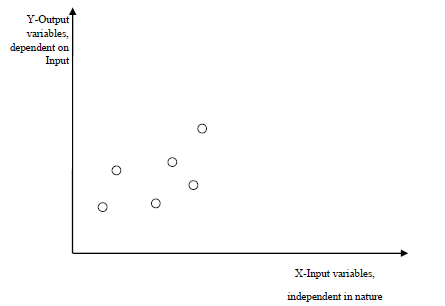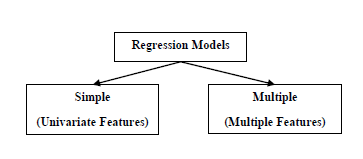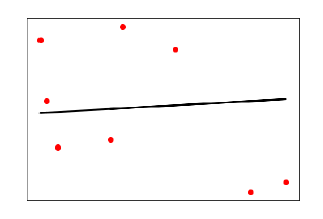# Regression Algorithms - Overview

## Introduction to Regression

Regression is another important and broadly used statistical and machine learning tool. The key objective of regression-based tasks is to predict output labels or responses which are continues numeric values, for the given input data. The output will be based on what the model has learned in training phase. Basically, regression models use the input data features (independent variables) and their corresponding continuous numeric output values (dependent or outcome variables) to learn specific association between inputs and corresponding outputs.## Types of Regression ModelsRegression models are of following two types −

Simple regression model − This is the most basic regression model in which predictions are formed from a single, univariate feature of the data.

Multiple regression model − As name implies, in this regression model the predictions are formed from multiple features of the data.

## Building a Regressor in Python

Regressor model in Python can be constructed just like we constructed the classifier. Scikit-learn, a Python library for machine learning can also be used to build a regressor in Python.

In the following example, we will be building basic regression model that will fit a line to the data i.e. linear regressor. The necessary steps for building a regressor in Python are as follows −

Step 1: Importing necessary python package

For building a regressor using scikit-learn, we need to import it along with other necessary packages. We can import the by using following script −

import numpy as np
from sklearn import linear_model
import sklearn.metrics as sm
import matplotlib.pyplot as plt


Step 2: Importing dataset

After importing necessary package, we need a dataset to build regression prediction model. We can import it from sklearn dataset or can use other one as per our requirement. We are going to use our saved input data. We can import it with the help of following script −

input = r'C:\linear.txt'


input_data = np.loadtxt(input, delimiter=',')
X, y = input_data[:, :-1], input_data[:, -1]


Step 3: Organizing data into training & testing sets

As we need to test our model on unseen data hence, we will divide our dataset into two parts: a training set and a test set. The following command will perform it −

training_samples = int(0.6 * len(X))
testing_samples = len(X) - num_training
X_train, y_train = X[:training_samples], y[:training_samples]
X_test, y_test = X[training_samples:], y[training_samples:]


Step 4: Model evaluation & prediction

After dividing the data into training and testing we need to build the model. We will be using LineaRegression() function of Scikit-learn for this purpose. Following command will create a linear regressor object.

reg_linear = linear_model.LinearRegression()


Next, train this model with the training samples as follows −

reg_linear.fit(X_train, y_train)


Now, at last we need to do the prediction with the testing data.

y_test_pred = reg_linear.predict(X_test)


Step 5: Plot & visualization

After prediction, we can plot and visualize it with the help of following script −

plt.scatter(X_test, y_test, color = 'red')
plt.plot(X_test, y_test_pred, color = 'black', linewidth = 2)
plt.xticks(())
plt.yticks(())
plt.show()


OutputIn the above output, we can see the regression line between the data points.

Step 6: Performance computation − We can also compute the performance of our regression model with the help of various performance metrics as follows.

print("Regressor model performance:")
print("Mean absolute error(MAE) =", round(sm.mean_absolute_error(y_test, y_test_pred), 2))
print("Mean squared error(MSE) =", round(sm.mean_squared_error(y_test, y_test_pred), 2))
print("Median absolute error =", round(sm.median_absolute_error(y_test, y_test_pred), 2))
print("Explain variance score =", round(sm.explained_variance_score(y_test, y_test_pred), 2))
print("R2 score =", round(sm.r2_score(y_test, y_test_pred), 2))


Output

Regressor model performance:
Mean absolute error(MAE) = 1.78
Mean squared error(MSE) = 3.89
Median absolute error = 2.01
Explain variance score = -0.09
R2 score = -0.09


## Types of ML Regression Algorithms

The most useful and popular ML regression algorithm is Linear regression algorithm which further divided into two types namely −

• Simple Linear Regression algorithm
• Multiple Linear Regression algorithm.

We will discuss about it and implement it in Python in the next chapter.

## Applications

The applications of ML regression algorithms are as follows −

Forecasting or Predictive analysis − One of the important uses of regression is forecasting or predictive analysis. For example, we can forecast GDP, oil prices or in simple words the quantitative data that changes with the passage of time.

Optimization − We can optimize business processes with the help of regression. For example, a store manager can create a statistical model to understand the peek time of coming of customers.

Error correction − In business, taking correct decision is equally important as optimizing the business process. Regression can help us to take correct decision as well in correcting the already implemented decision.

Economics − It is the most used tool in economics. We can use regression to predict supply, demand, consumption, inventory investment etc.

Finance − A financial company is always interested in minimizing the risk portfolio and want to know the factors that affects the customers. All these can be predicted with the help of regression model.